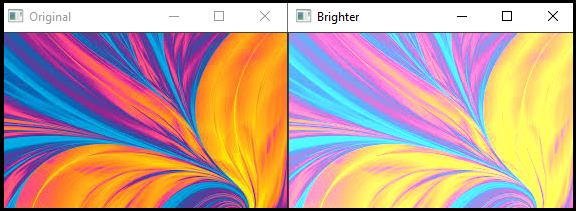# How to change the brightness of an image in OpenCV using C++?

Changing the brightness means changing the value of pixels. It means adding or subtracting value some integer value with the current value of each pixel. When you add some integer value with every pixel, it means you are making the image brighter. When you subtract some constant value from all of the pixels, you are reducing the brightness. First, we will learn how to increase the brightness and second we will learn how to reduce the brightness.

## Increasing the Brightness

Increasing the Brightness using OpenCV is very easy. To increase the brightness, add some additional values with each channel, and the brightness will be increased. For example, BRG images have three channels blue (B), green (G) and red(R). That means the current value of a pixel will be (B. G, R). To increase the brightness, we have to add some scalar number with it such as (B, G, R) + (10, 10, 10) or (B, G, R) + (20, 20, 20) or whatever number you want.

The following example performs the image brightening −

## Example

#include<iostream>
#include<opencv2/highgui/highgui.hpp>
using namespace cv;
using namespace std;
int main() {
Mat original;//Declaring a matrix to load the original image//
Mat brighter;//Declaring a matrix to load the image after changing the brightness//
namedWindow("Original");//Declaring window to show the original image//
namedWindow("Brighter");//Declaring window to show the brighter image//
}